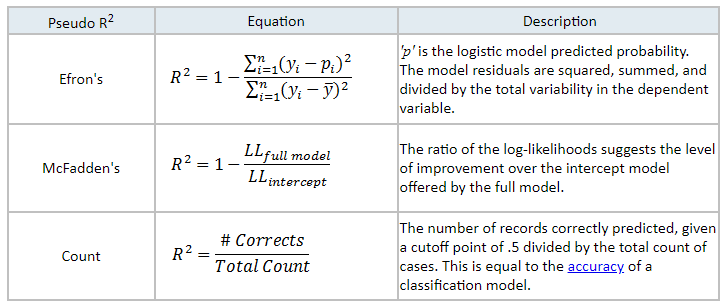## 8.1 Pseudo $$R^2$$

• Unlike linear regression models, logistic models do not use or have an equivalent to $$R^2$$ that exactly determines the variance explained by the model.

• Different methods have been developed known as \$$$operatorname{pseudo}-R^2$$ that attempt to approximate the concept of the coefficient of determination. They range from 0 to 1, but by no means can they be interpreted as the same as $$R^2$$.

One of the $$\operatorname{pseudo}-R^2$$ for logistic regression was proposed by McFadden’s:

$R^{2}_{\text{McFadden}} = 1- \frac{\log(L(M_1))}{\log(L(M_0))},$

where $$L$$ is the value of the likelihood of each model. In this case, $$\log(L)$$, has a meaning analogous to the sum of squares of linear regression. Hence it is called $$\operatorname{pseudo}-R^2$$.

$$R^{2}_{\text{McFadden}}$$ is equals to$$0$$ if the fitted model does not improve on the null model, and a value of $$1$$ if it fits the data perfectly.

• In our example:

$\text{McFadden } R^2 = 0.142147,$

Other pseudo-$$R2$$: